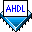## Setting Clock, Reset & Enable Signals

 See AlsoClock, reset, and clock enable signals control the flipflops of the state register in the state machine. These signals are specified with Boolean control equations in the Logic Section.

In the file simple.tdf shown below, the state machine clock is driven by the input `clk`. The state machine's asynchronous reset signal is driven by `reset`, which is active high. In this design file, the declaration of the `ena` input in the Subdesign Section and the Boolean control equation `ss.ena = ena` in the Logic Section connect the clock enable signal.

```SUBDESIGN simple
(
clk, reset, ena, d : INPUT;
q                  : OUTPUT;
)
VARIABLE
ss: MACHINE WITH STATES (s0, s1);
BEGIN
ss.clk = clk;
ss.reset = reset;
ss.ena = ena;

CASE ss IS
WHEN s0 =>
q = GND;

IF d THEN
ss = s1;
END IF;
WHEN s1 =>
q = VCC;

IF !d THEN
ss = s0;
END IF;
END CASE;
END;
```